Home

# Artinianness of Composed Graded Local Cohomology Modules

## Abstract

Let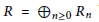$R\,=\,{{\oplus }_{n\ge 0}}{{R}_{n}}$ be a graded Noetherian ring with local base ring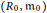$\left( {{R}_{0}},{{\text{m}}_{0}} \right)$ and let${{R}_{+}}\,=\,{{\oplus }_{n>0}}{{R}_{n}}$ . Let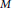$M$ and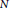$N$ be finitely generated graded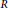$R$ -modules and let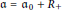$\mathfrak{a}\,=\,{{\mathfrak{a}}_{0}}\,+\,{{R}_{+}}$ an ideal of$R$ . We show that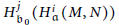$H_{\mathfrak{b}0}^{j}\,\left( H_{\mathfrak{a}}^{i}\left( M,\,N \right) \right)$ and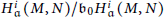${H_{\mathfrak{a}}^{i}\left( M,\,N \right)}/{{{\mathfrak{b}}_{0}}H_{\mathfrak{a}}^{i}\left( M,\,N \right)}\;$ are Artinian for some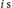$i\text{ s}$ and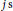$j\,\text{s}$ with a specified property, where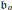${{\mathfrak{b}}_{o}}$ is an ideal of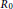${{R}_{0}}$ such that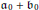${{\mathfrak{a}}_{0}}\,+\,{{\mathfrak{b}}_{0}}$ is an${{\mathfrak{m}}_{0}}$ -primary ideal.

## References

Hide All
MathJax
MathJax is a JavaScript display engine for mathematics. For more information see http://www.mathjax.org.

# Artinianness of Composed Graded Local Cohomology Modules

## Metrics

### Full text viewsFull text views reflects the number of PDF downloads, PDFs sent to Google Drive, Dropbox and Kindle and HTML full text views.

Total number of HTML views: 0
Total number of PDF views: 0 *Loading metrics...

### Abstract viewsAbstract views reflect the number of visits to the article landing page.

Total abstract views: 0 *Loading metrics...

* Views captured on Cambridge Core between <date>. This data will be updated every 24 hours.

Usage data cannot currently be displayed